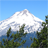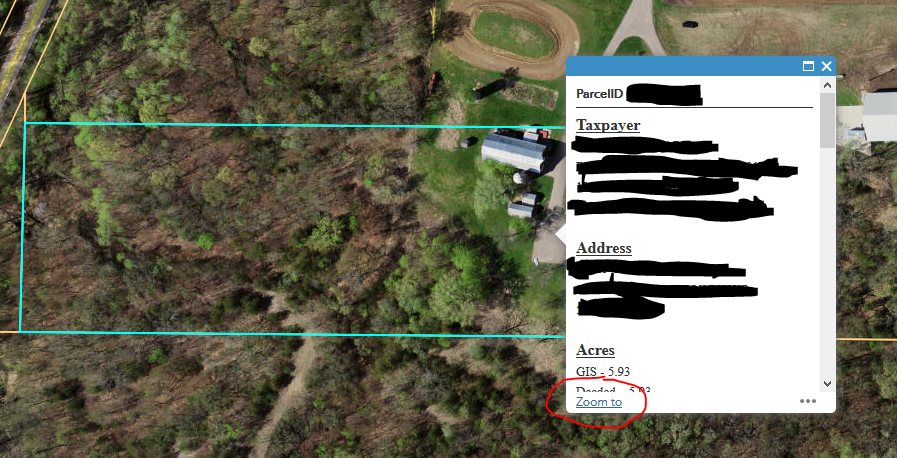# Search Widget - Zoom to result is too close, is there a way to customize it?

3923
12
Jump to solution
04-21-2016 06:06 PMNew Contributor III

Hi All,

I have a search widget and it's using our own layer source (feature service, polygon). When I click the search result it will zoom to the selected polygon extent and the polygon occupied the whole map content screen. Can the search result not to zoom in too close? is there anyway to customize it?

thanks,

Wilson

Tags (4)
1 Solution

Accepted SolutionsbyMVP Esteemed Contributor

Wilson,

So it looks like you are using minified code (so you must have downloaded this from AGOL). In that case then the code would be:

`_onSelectResult: function(b) {            var c = b.result;            if (c && c.name) {                var h = b.sourceIndex;                b = this.searchResults;                for (var m = 0, r = 0, t = b.length; r < t; r++)                    if (l.isEqual(b, c)) {                        m = r;                        break                    }                p("li", this.searchResultsNode).forEach(n.hitch(this, function(b) {                    q.removeClass(b, "result-item-selected");                    var l = q.getAttr(b, "title"),                        n = q.getAttr(b, "data-index"),                        p = q.getAttr(b, "data-source-index");                    l === c.name && (n === m.toString() && p === h.toString()) && q.addClass(b, "result-item-selected")                }))            }            var pt = c.feature.geometry.getCentroid();             this.map.centerAndZoom(pt, 2); //Change this to suit your needs        },`
12 RepliesbyMVP Esteemed Contributor

Wilson,

Sure, if you add two lines to the search widgets Widget.js _onSelectResult function (lines 29 and 30):

`_onSelectResult: function(e) {        var result = e.result;        if (!(result && result.name)) {          return;        }        var dataSourceIndex = e.sourceIndex;        var sourceResults = this.searchResults[dataSourceIndex];        var dataIndex = 0;        for (var i = 0, len = sourceResults.length; i < len; i++) {          if (jimuUtils.isEqual(sourceResults, result)) {            dataIndex = i;            break;          }        }        query('li', this.searchResultsNode)          .forEach(lang.hitch(this, function(li) {            html.removeClass(li, 'result-item-selected');            var title = html.getAttr(li, 'title');            var dIdx = html.getAttr(li, 'data-index');            var dsIndex = html.getAttr(li, 'data-source-index');            if (title === result.name &&              dIdx === dataIndex.toString() &&              dsIndex === dataSourceIndex.toString()) {              html.addClass(li, 'result-item-selected');            }          }));          var pt = result.feature.geometry.getCentroid();          this.map.centerAndZoom(pt, 2); //Change this to suit your needs      },`New Contributor III

Hi Robert,

thank you : )

However, I couldn't find your exact codes in the Search/Widget.js

These are the closet codes I found. May I ask where to insert those 2 lines?

Best,

Wilson

_onSelectResult: function(b) {

var c = b.result;

if (c && c.name) {

var h = b.sourceIndex;

b = this.searchResults;

for (var m = 0, r = 0, t = b.length; r < t; r++)

if (l.isEqual(b, c)) {

m = r;

break

}

p("li", this.searchResultsNode).forEach(n.hitch(this, function(b) {

q.removeClass(b, "result-item-selected");

var l = q.getAttr(b, "title"),

n = q.getAttr(b, "data-index"),

p = q.getAttr(b, "data-source-index");

l === c.name && (n === m.toString() && p === h.toString()) && q.addClass(b, "result-item-selected")

}))

}

},byMVP Esteemed Contributor

Wilson,

So it looks like you are using minified code (so you must have downloaded this from AGOL). In that case then the code would be:

`_onSelectResult: function(b) {            var c = b.result;            if (c && c.name) {                var h = b.sourceIndex;                b = this.searchResults;                for (var m = 0, r = 0, t = b.length; r < t; r++)                    if (l.isEqual(b, c)) {                        m = r;                        break                    }                p("li", this.searchResultsNode).forEach(n.hitch(this, function(b) {                    q.removeClass(b, "result-item-selected");                    var l = q.getAttr(b, "title"),                        n = q.getAttr(b, "data-index"),                        p = q.getAttr(b, "data-source-index");                    l === c.name && (n === m.toString() && p === h.toString()) && q.addClass(b, "result-item-selected")                }))            }            var pt = c.feature.geometry.getCentroid();             this.map.centerAndZoom(pt, 2); //Change this to suit your needs        },`New Contributor III

thank you so much!

I will give it a trybyMVP Esteemed Contributor

Don't forget to mark the question as answered by clicking on the "Correct Answer" link for the rely that answered your question.New Contributor III

Hi all,

I have a question similar to the one posted by Wilson.  The code that Robert provided above works great, however I am searching by parcels in my app and the actual size of each parcel polygon can very greatly.  Having a set extent for all parcels being searched either results in the zoom extent being too far in for large parcels or too far out for smaller parcels.  I have noticed that when I search for a parcel and then click on the Zoom to link on the bottom right hand side of the popup (see following screenshot), the zoom extent goes to the best fit cache scale.  Is there anyway to have this be the default in the Search widget?  I have been playing around with code some but cannot get it to work correctly.Thanks in advance,

CraigbyMVP Esteemed Contributor

Craig,

For that you can just get the extent of the polygon and set the maps extent to that.

(change those two lines to):

``          var ext = result.feature.geometry.getExtent();            this.map.setExtent(ext, true);‍‍``

Of course you will want to put some code in there to verify that the geometry type is actually a polygon.New Contributor III

Robert,

Thanks again, this is exactly what I was looking for.

CraigRegular Contributor

I tried add the two lines but i get "Create Widget Error". ?

I am on WAB 2.7

if (result &&
result.name &&
title === result.name.toString() &&
dIdx === dataIndex.toString() &&
dsIndex === dataSourceIndex.toString()) {
html.addClass(li, 'result-item-selected');
}
}));
var pt = result.feature.geometry.getCentroid();
this.map.centerAndZoom(pt, 2); //Change this to suit your needs
},
//var layer = this.map.getLayer(sourceLayerId);
var layerInfo = this.layerInfosObj.getLayerInfoById(sourceLayerId);